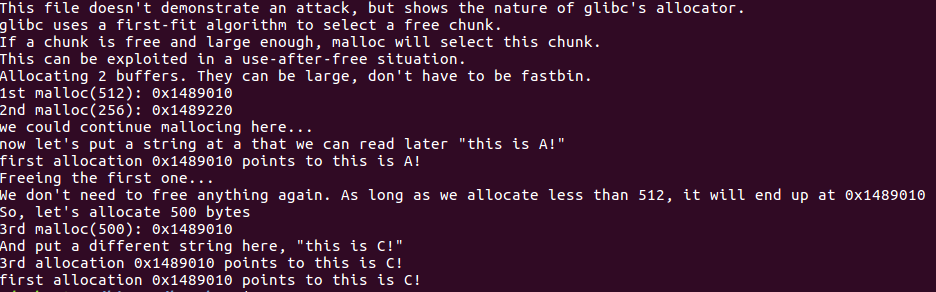# 0x00 Introduction

how2heap 是一个堆利用技术系列教程，我会将这个教程的学习过程记录下来。

# 0x01 Overview

``````#include <stdio.h>
#include <stdlib.h>
#include <string.h>

int main()
{
fprintf(stderr, "This file doesn't demonstrate an attack, but shows the nature of glibc's allocator.\n");
fprintf(stderr, "glibc uses a first-fit algorithm to select a free chunk.\n");
fprintf(stderr, "If a chunk is free and large enough, malloc will select this chunk.\n");
fprintf(stderr, "This can be exploited in a use-after-free situation.\n");

fprintf(stderr, "Allocating 2 buffers. They can be large, don't have to be fastbin.\n");
char* a = malloc(512);
char* b = malloc(256);
char* c;

fprintf(stderr, "1st malloc(512): %p\n", a);
fprintf(stderr, "2nd malloc(256): %p\n", b);
fprintf(stderr, "we could continue mallocing here...\n");
fprintf(stderr, "now let's put a string at a that we can read later \"this is A!\"\n");
strcpy(a, "this is A!");
fprintf(stderr, "first allocation %p points to %s\n", a, a);

fprintf(stderr, "Freeing the first one...\n");
free(a);

fprintf(stderr, "We don't need to free anything again. As long as we allocate less than 512, it will end up at %p\n", a);

fprintf(stderr, "So, let's allocate 500 bytes\n");
c = malloc(500);
fprintf(stderr, "3rd malloc(500): %p\n", c);
fprintf(stderr, "And put a different string here, \"this is C!\"\n");
strcpy(c, "this is C!");
fprintf(stderr, "3rd allocation %p points to %s\n", c, c);
fprintf(stderr, "first allocation %p points to %s\n", a, a);
fprintf(stderr, "If we reuse the first allocation, it now holds the data from the third allocation.");
}
``````

# 0x02 First-fit behavior

``````char *a = malloc(300);    // 0x***010
char *b = malloc(250);    // 0x***150

free(a);

a = malloc(250);          // 0x***010
``````

unsorted bin 的变化为：

1. 释放 ‘a’。

2. ‘malloc’ 请求。

head -> a2 -> tail [ ‘a1’ 被返回了 ]

fast chunk 的情况也是如此。不过释放后不是放入 unsorted bin，而是放入 fastbin 中。就跟之前提到的一样，fastbin 存储了一个插入和删除都是在头部的单链表，反转了 chunk 获取后的顺序。

``````char *a = malloc(20);     // 0xe4b010
char *b = malloc(20);     // 0xe4b030
char *c = malloc(20);     // 0xe4b050
char *d = malloc(20);     // 0xe4b070

free(a);
free(b);
free(c);
free(d);

a = malloc(20);           // 0xe4b070
b = malloc(20);           // 0xe4b050
c = malloc(20);           // 0xe4b030
d = malloc(20);           // 0xe4b010
``````

fastbin 的变化：

1. ‘a’ freed.

2. ‘b’ freed.

head -> b -> a -> tail

3. ‘c’ freed.

head -> c -> b -> a -> tail

4. ‘d’ freed.

head -> d -> c -> b -> a -> tail

5. ‘malloc’ request.

head -> c -> b -> a -> tail [ ‘d’ is returned ]

6. ‘malloc’ request.

head -> b -> a -> tail [ ‘c’ is returned ]

7. ‘malloc’ request.

head -> a -> tail [ ‘b’ is returned ]

8. ‘malloc’ request.

head -> tail [ ‘a’ is returned ]

first_fit 运行截图：